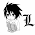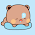Thursday, February 28, 2013

Euler Totient / φ Function

Euler Totient / Phi Function φ(n) counts the number of positive integers less than or equal to n which are relatively prime to n, i.e. do not have any common divisor with n except 1.

Formula for Euler Phi function:

Here, the product is over the distinct prime numbers which divide n. Now, you can just factorize n and calculate φ(n) pretty easily. But, will that be efficient for a task such as you are asked to find φ(n) over a range of integers?

If you look closely to the formula, you will see that, we multiply n with (p-1)/p for each prime p that divides n. Now recall what do we do when we run Sieve of Eratosthenes for marking primes / non-primes. On the outer loop of the sieve, we determine if a number is prime, then in inner loop, instead of setting flags, if we can keep multiplying the number with (p-1)/p where p is the current prime number from outer loop, at the end of the iterations, we can actually generate φ(n) for each n over the range we are asked for. Here is a source code example that does exactly the same thing. Just take a look and try to understand what are the loops doing here, and how we are performing calculation and storing results.

```#include <cstdio>

const int MAX = 1000001;
int phi[MAX];

void euler_phi() {
phi = 1;
for(int i=2; i<MAX; i++) {
if(!phi[i]) {
phi[i] = i-1;
for(int j=(i<<1); j<MAX; j+=i) {
if(!phi[j]) phi[j] = j;
phi[j] = phi[j]/i*(i-1);
}
}
}
}

int main() {
euler_phi();
for(int t=1; t<MAX; t++) printf("%d\n", phi[t]);
return 0;
}
```

A bit of explanation on what we are doing here: Initially the phi[] array is set to 0 (as it is declared global). We know that, phi = 1 and phi[n] = n-1 when n is a prime number. So, similar to sieve algorithm, first we check if current number is prime in the outer loop, if phi[i] = 0, it means i is prime. So, we update it with i-1 accordingly. Now, for all the multiples of i greater than i, which starts from 2*i, calling it j in inner loop, we need to multiply phi[j] by (i-1) / i. Here, we first check if phi[j] is 0, i.e. visiting it for the first time, in this case we set it with j, as I said earlier that, for φ(n) we will multiply n with (p-1)/p where p are the primes that divide n. Also, one thing to note here: a * b / c and a / c * b are not always same for integer calculation unless you can assure that c divides a. In this case it does, why? cause this is basically a prime factoring algorithm, and c = i here. As we are traversing i's multiples, it is guaranteed that a=phi[j] can be divided by c=i and instead of a * b / c format, we will always use a / c * b in these types of situations, because it will help preventing overflow many times.

Now, think about the optimizations we could apply here, and try applying them, like discarding even numbers, starting inner loop from squares, increment inner loop twice the prime number each time, won't work here. Because, we need to go through every numbers in inner loop, as we are trying to find Totient function for every n in the range 1 to MAX. Test the code on smaller range, and try to check if it is doing this correctly, play around with it.

1.1.